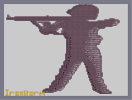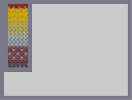#Hover over the thumbnail for a full-size version.

Author ironstorm author:ironstorm n-art nonplayable rated 2008-09-08 2008-09-08 4 by 12 people. \$Paint it Black, Please.#ironstorm#none#00000000000000000000000000000000000000000000000000000000000000000000000000000000000000000000000000000000000000000000000000000000000000000000000000000000000000000000000000000000000000000000000000000000000000000000000000000000000000000000000000000000000000000000000000000000000000000000000000000000000000000000000000000000000000000000000000000000000000000000000000000000000000000000000000000000000000000000000000000000000000000000000000000000000000000000000000000000000000000000000000000000000000000000000000000000000000000000000000000000000000000000000000000000000000000000000000000000000000000000000000000000000000000000000000000000000000000000000000000000000000000000000000000000000000000000000000000000000000000|10^42,114!10^54,114!10^48,114!10^60,114!10^66,114!10^72,114!10^78,114!10^84,114!3^42,108!3^48,108!3^54,108!3^60,108!3^66,108!3^72,108!3^78,108!3^84,108!3^90,102!3^96,102!3^102,102!3^108,102!3^114,102!3^120,102!3^126,102!3^132,102!3^138,102!3^144,102!3^150,102!3^156,102!3^162,102!3^162,102!3^168,102!3^174,102!3^180,102!3^186,102!3^192,102!3^198,102!3^204,102!3^210,102!3^216,102!3^222,102!3^228,102!3^234,102!3^240,102!3^246,102!3^252,102!3^258,102!3^264,102!3^270,102!3^276,102!3^282,102!3^282,102!3^288,102!3^294,102!3^300,102!3^306,102!3^312,102!3^318,102!3^324,102!3^324,102!3^330,102!3^336,102!3^342,102!3^348,102!3^354,102!3^360,102!3^366,102!3^372,102!3^378,102!3^384,102!3^396,102!3^402,102!3^390,102!3^408,102!3^414,102!3^420,102!3^426,102!3^90,108!3^96,108!3^102,108!3^114,108!3^108,108!3^120,108!3^126,108!3^132,108!3^138,108!3^144,108!3^156,108!3^150,108!3^162,108!3^168,108!3^174,108!3^180,108!3^186,108!3^192,108!3^198,108!3^204,108!3^210,108!3^216,108!3^222,108!3^228,108!3^234,108!3^240,108!3^246,108!3^252,108!3^258,108!3^264,108!3^276,108!3^282,108!3^270,108!3^288,108!3^294,108!3^300,108!3^312,108!3^306,108!3^318,108!3^324,108!3^330,108!3^336,108!3^342,108!3^348,108!3^354,108!3^360,108!3^366,108!3^372,108!3^378,108!3^384,108!3^390,108!3^396,108!3^402,108!3^414,108!3^408,108!3^420,108!3^414,108!3^426,108!3^90,114!3^96,114!3^102,114!3^108,114!3^114,114!3^120,114!3^126,114!3^132,114!3^138,114!3^144,114!3^150,114!3^156,114!3^162,114!3^168,114!3^174,114!3^180,114!3^186,114!3^192,114!3^198,114!3^204,114!3^210,114!3^216,114!3^222,114!3^228,114!3^234,114!3^240,114!3^246,114!3^252,114!3^258,114!3^264,114!3^270,114!3^276,114!3^282,114!3^288,114!3^294,114!3^300,114!3^306,114!3^312,114!3^318,114!3^324,114!3^330,114!3^336,114!3^342,114!3^348,114!3^354,114!3^360,114!3^372,114!3^366,114!3^378,114!3^384,114!3^390,114!3^396,114!3^402,114!3^408,114!3^414,114!3^420,114!3^426,114!10^90,120!10^96,120!10^102,120!10^108,120!10^114,120!10^120,120!10^126,120!10^132,120!10^138,120!10^144,120!10^150,120!10^156,120!10^162,120!10^168,120!10^174,120!10^180,120!10^186,120!10^192,120!10^198,120!10^204,120!10^210,120!10^216,120!10^228,120!10^222,120!10^234,120!10^240,120!10^246,120!10^252,120!10^264,120!10^258,120!3^48,102!3^48,96!3^48,90!3^54,96!3^60,102!10^270,120!10^276,120!10^282,120!10^288,120!10^294,120!10^300,120!10^306,120!10^312,120!10^318,120!10^324,120!10^330,120!10^336,120!10^342,120!10^348,120!10^354,120!10^360,120!10^366,120!10^372,120!10^378,120!10^384,120!10^390,120!10^396,120!10^402,120!10^408,120!10^414,120!10^420,120!10^426,120!3^282,96!3^288,96!10^186,126!10^192,132!10^198,138!10^204,144!10^210,144!10^216,150!10^222,156!10^228,156!10^234,162!10^234,168!10^240,168!10^240,174!10^240,180!10^240,186!10^246,192!10^252,198!10^258,198!10^264,204!10^270,204!10^276,204!10^282,204!10^288,204!10^294,198!10^300,198!10^306,198!10^312,198!10^318,198!10^324,198!10^330,198!10^324,204!10^330,204!10^336,204!10^342,204!10^348,204!10^354,204!10^192,126!10^198,132!10^204,138!10^210,138!10^216,144!10^222,150!10^234,156!10^240,162!10^246,168!10^246,174!10^246,180!10^246,186!10^252,192!10^258,192!10^264,192!10^264,198!10^270,198!10^276,198!10^282,198!10^288,198!10^288,192!10^294,192!10^300,192!10^306,192!10^312,192!10^318,192!10^324,192!10^330,192!10^336,192!10^336,198!10^342,198!10^348,198!3^198,126!3^204,126!3^210,126!3^216,126!3^204,132!3^210,132!3^216,132!3^216,138!3^222,144!3^228,150!3^240,156!3^234,150!3^228,144!3^222,138!3^246,162!3^252,162!3^258,162!3^264,162!3^264,156!3^264,150!3^270,150!3^270,144!3^270,138!3^270,132!3^264,132!3^258,132!3^252,132!3^246,132!3^240,132!3^234,132!3^228,132!3^324,186!3^324,180!3^324,174!3^330,174!3^336,174!3^336,168!3^336,162!3^348,162!3^342,162!3^348,156!3^348,150!3^354,150!3^276,132!3^282,132!3^288,132!3^294,132!3^294,138!3^300,138!3^300,144!3^300,150!3^306,150!3^312,150!3^318,150!3^318,144!3^324,144!3^330,138!3^336,138!3^342,132!3^252,168!3^252,174!3^252,180!3^258,180!3^264,180!3^270,186!3^276,186!3^282,186!3^294,186!3^300,186!3^288,186!3^306,186!3^312,186!3^312,180!3^312,174!3^324,162!3^336,156!3^336,150!3^342,144!3^330,150!3^330,156!3^324,156!3^330,168!3^324,168!3^318,168!3^318,162!3^312,156!3^300,156!3^294,156!3^294,150!3^294,144!3^288,144!3^282,138!3^282,144!3^276,144!3^276,156!3^282,156!3^270,156!3^282,156!3^288,156!3^306,162!3^312,168!3^306,168!3^300,168!3^294,168!3^288,168!3^282,168!3^276,168!3^270,168!3^264,168!3^258,174!3^270,174!3^276,174!3^282,174!3^288,180!3^300,180!3^294,180!3^294,174!3^264,186!3^264,180!3^294,162!3^288,162!3^282,162!3^276,162!3^270,180!3^276,180!3^264,174!3^282,150!3^276,186!3^300,174!3^306,162!3^288,174!3^306,156!3^312,156!3^306,168!3^318,162!3^324,150!3^276,192!3^270,192!3^282,192!3^252,186!3^258,186!3^252,180!3^282,186!3^282,180!3^306,180!3^312,180!3^318,180!3^324,180!3^324,174!3^318,174!3^312,174!3^306,174!3^324,186!3^324,186!3^318,186!3^300,162!3^306,162!3^318,162!3^312,162!3^318,162!3^318,162!3^324,162!3^318,156!10^252,186!10^270,192!3^360,150!3^354,162!3^336,168!3^342,150!3^336,186!3^330,186!3^342,180!3^342,174!3^348,174!3^354,168!3^360,162!3^348,132!3^360,132!3^366,132!3^366,138!3^366,150!3^366,162!3^360,138!3^354,138!3^348,138!3^366,150!3^366,156!3^354,144!3^342,144!10^360,204!10^366,204!3^432,96!3^432,90!3^426,84!3^420,78!3^414,78!3^408,78!3^408,78!10^408,78!10^414,78!10^420,78!10^402,72!10^408,66!10^414,60!10^420,60!10^426,60!10^432,54!10^438,48!10^444,42!10^450,42!10^456,36!10^462,36!10^468,36!10^474,30!3^480,30!3^486,30!3^492,30!3^498,30!3^504,30!3^510,30!3^516,30!3^522,30!3^528,30!3^534,30!3^540,30!3^546,30!3^552,30!3^510,24!3^516,24!3^552,36!3^558,36!3^564,36!3^570,42!3^576,42!3^564,42!3^576,48!3^582,48!3^588,54!3^588,60!3^588,66!3^588,72!3^588,78!3^582,78!3^582,84!3^582,90!3^582,96!3^576,96!3^576,102!3^576,108!3^588,126!3^594,126!3^600,126!3^606,126!3^570,114!3^564,114!3^558,114!3^552,114!3^546,114!3^540,114!3^534,114!3^528,114!3^528,126!3^534,132!3^528,132!3^540,132!3^546,132!3^552,132!3^558,132!3^564,132!3^570,132!3^576,132!3^582,132!3^588,132!3^612,126!3^618,126!3^624,126!3^630,126!3^630,120!3^636,120!3^642,120!3^648,120!3^654,120!3^660,114!3^660,120!3^666,114!3^672,114!3^678,114!3^684,114!3^684,120!3^690,120!3^696,120!3^702,120!3^708,120!3^708,126!3^708,132!3^708,138!3^702,144!3^696,150!3^690,150!3^684,150!3^678,150!3^672,156!3^672,150!3^666,156!3^660,156!3^654,156!3^648,156!3^642,156!3^636,156!3^630,162!3^624,168!3^618,168!3^612,168!3^606,168!3^606,168!3^600,168!3^594,168!3^594,174!3^588,180!3^582,186!3^582,192!3^582,198!3^582,204!3^714,126!3^714,132!3^582,180!3^582,174!3^588,168!3^582,210!3^588,216!3^582,216!3^594,216!3^600,216!3^606,222!3^612,228!3^612,234!3^606,240!3^600,240!3^600,246!3^600,252!3^594,252!3^594,258!3^594,264!3^582,264!3^588,264!3^576,264!3^576,270!3^576,276!3^576,282!3^582,288!3^588,294!3^594,300!3^576,288!3^582,294!3^588,300!3^594,306!3^594,312!3^594,318!3^600,318!3^600,324!3^600,330!3^600,336!3^606,336!3^606,342!3^606,348!3^606,354!3^606,360!3^606,366!3^606,372!3^606,378!3^606,384!3^606,396!3^606,390!3^606,402!3^606,408!3^606,414!3^606,420!3^606,426!3^606,432!3^612,438!3^612,444!3^612,450!3^612,456!3^606,438!3^606,444!3^606,450!3^606,456!3^606,462!3^606,468!3^606,474!3^612,474!3^612,480!3^618,480!3^618,486!3^618,492!3^618,498!3^624,504!3^630,510!3^636,516!3^636,522!3^636,528!3^636,534!3^636,540!3^642,540!3^648,540!3^654,546!3^648,546!3^654,552!3^654,558!3^648,558!3^666,558!3^666,552!3^660,552!3^660,558!3^642,558!3^636,558!3^630,558!3^624,558!3^618,558!3^612,558!3^606,558!3^276,162!3^258,174!3^600,558!3^600,552!3^594,552!3^588,552!3^588,546!3^582,546!3^576,546!3^576,540!3^570,540!3^564,540!3^564,534!3^558,534!3^558,528!3^558,522!3^558,510!3^558,516!3^552,510!3^552,504!3^552,498!3^552,492!3^546,492!3^546,486!3^546,480!3^540,480!3^540,474!3^540,468!3^540,462!3^534,462!3^534,456!3^534,450!3^534,444!3^528,444!3^528,438!3^528,432!3^528,426!3^522,426!3^522,420!3^522,414!3^522,408!3^522,402!3^516,402!3^516,396!3^516,390!3^516,384!3^510,384!3^510,378!3^504,372!3^498,366!3^492,366!3^486,372!3^480,378!3^480,384!3^474,390!3^468,396!3^468,402!3^462,408!3^456,408!3^456,414!3^456,420!3^450,426!3^444,432!3^444,438!3^438,444!3^432,450!3^432,444!3^450,420!3^426,456!3^426,462!3^426,468!3^420,468!3^420,474!3^414,474!3^408,480!3^408,486!3^408,492!3^402,498!3^396,504!3^390,510!3^384,516!3^384,510!3^390,504!3^396,498!3^384,522!3^378,528!3^372,534!3^366,540!3^354,540!3^360,540!3^348,540!3^342,540!3^336,540!3^336,546!3^330,546!3^324,546!3^318,546!3^318,552!3^312,552!3^306,552!3^300,552!3^294,552!3^288,552!3^282,552!3^276,552!3^270,552!3^264,552!3^258,552!3^258,546!3^264,540!3^270,540!3^276,534!3^282,534!3^288,534!3^294,534!3^300,534!3^300,528!3^300,522!3^300,516!3^312,516!3^306,516!3^312,510!3^312,504!3^312,498!3^318,492!3^324,486!3^330,486!3^336,486!3^336,480!3^336,474!3^336,468!3^336,462!3^336,456!3^342,456!3^348,450!3^354,444!3^360,444!3^360,432!3^360,438!3^360,426!3^360,420!3^360,414!3^366,414!3^372,414!3^372,408!3^372,402!3^372,396!3^378,396!3^384,390!3^384,378!3^384,384!3^384,372!3^390,366!3^396,366!3^396,360!3^396,354!3^396,348!3^396,336!3^396,342!3^396,330!3^396,324!3^402,318!3^402,312!3^402,306!3^402,300!3^402,294!3^402,288!3^402,282!3^402,276!3^402,270!3^402,264!3^402,258!3^402,246!3^402,240!3^402,234!3^402,252!3^402,228!3^396,228!3^390,222!3^390,216!3^390,210!3^384,210!3^378,204!3^372,204!3^372,198!3^372,192!3^372,186!3^378,186!3^378,180!3^378,174!3^378,162!3^378,168!3^378,156!3^378,150!3^378,144!3^378,132!3^378,138!3^372,132!3^372,138!3^372,144!3^372,150!3^372,156!3^372,162!3^360,150!3^348,156!3^342,162!3^342,150!3^348,144!3^342,144!3^336,144!3^336,156!3^336,162!3^348,156!3^354,150!3^360,144!3^342,126!3^348,126!3^354,126!3^360,126!3^366,126!3^378,126!3^336,132!10^258,546!10^264,540!10^270,540!10^276,534!10^282,534!10^288,534!10^294,534!10^300,534!10^300,528!10^300,522!10^300,516!10^306,516!10^312,516!10^312,510!10^312,504!10^312,498!10^318,492!10^324,486!10^330,486!10^336,486!10^336,480!10^336,474!10^336,468!10^336,462!10^336,456!10^342,456!10^348,450!10^354,444!10^360,444!10^360,438!10^360,432!10^360,426!10^360,420!10^360,414!10^366,414!10^372,414!10^372,408!10^372,402!10^372,396!10^378,396!10^384,390!10^384,384!10^384,378!10^384,372!10^390,366!10^396,366!10^396,354!10^396,360!10^396,348!10^396,342!10^396,336!10^396,330!10^396,324!10^402,318!10^402,312!10^402,306!10^402,300!10^402,294!10^402,288!10^402,282!10^402,276!10^402,270!10^402,264!10^402,258!10^402,252!10^402,246!10^402,240!10^402,234!10^402,228!10^396,228!10^390,222!10^390,216!10^390,210!10^384,210!10^378,204!10^372,204!10^414,72!10^420,66!10^432,66!10^426,66!10^438,60!10^438,54!10^444,54!10^450,48!10^462,42!10^456,48!10^468,42!10^480,42!10^480,36!10^474,42!10^450,54!10^426,72!10^492,36!10^480,30!10^486,30!10^486,36!10^270,546!10^276,546!10^276,540!10^282,540!10^288,540!10^294,540!10^300,540!10^306,540!10^306,534!10^306,528!10^306,522!10^312,522!10^318,522!10^318,516!10^318,510!10^318,504!10^318,498!10^324,498!10^324,492!10^330,492!10^336,492!10^342,492!10^342,486!10^342,480!10^342,474!10^342,468!10^342,462!10^348,462!10^348,456!10^354,456!10^360,450!10^366,450!10^366,444!10^366,438!10^366,432!10^366,426!10^366,420!10^372,420!10^378,420!10^378,414!10^378,408!10^378,402!10^384,402!10^390,402!10^390,396!10^390,390!10^390,384!10^390,378!10^390,372!10^396,372!10^402,372!10^402,366!10^402,360!10^402,354!10^402,348!10^402,342!10^402,336!10^402,330!10^402,324!10^408,324!10^408,318!10^408,312!10^408,306!10^408,300!10^408,288!10^408,294!10^408,282!10^408,276!10^408,270!10^408,264!10^408,258!10^408,252!10^408,246!10^408,240!10^408,234!10^408,228!10^408,222!10^402,222!10^396,222!10^396,216!10^396,210!10^396,204!10^390,204!10^384,204!10^384,198!10^378,198!10^288,546!10^294,546!10^300,546!10^306,546!10^312,546!10^312,540!10^312,534!10^318,534!10^324,534!10^324,528!10^324,522!10^324,516!10^324,510!10^324,504!10^330,504!10^330,498!10^336,498!10^342,498!10^348,498!10^348,492!10^348,486!10^348,480!10^348,474!10^354,468!10^360,462!10^366,456!10^372,456!10^372,450!10^372,444!10^372,438!10^372,432!10^372,426!10^378,426!10^384,426!10^384,420!10^384,414!10^390,426!10^390,420!10^390,414!10^384,408!10^396,408!10^396,402!10^396,396!10^396,390!10^396,384!10^396,378!10^402,378!10^408,378!10^408,372!10^408,366!10^408,360!10^408,348!10^408,342!10^408,330!10^408,336!10^408,354!10^414,324!10^414,318!10^414,312!10^414,306!10^414,300!10^348,468!10^360,456!10^414,294!10^414,288!10^414,282!10^414,276!10^414,270!10^414,264!10^414,258!10^414,246!10^414,252!10^414,234!10^414,228!10^414,240!10^414,222!10^414,216!10^408,216!10^402,216!10^402,210!10^402,204!10^402,198!10^396,198!10^390,198!10^390,192!10^384,192!10^408,72!10^474,36!10^420,78!10^420,66!10^414,72!10^420,72!10^432,60!10^414,66!10^456,42!10^444,48!3^324,540!3^330,540!3^330,534!3^330,528!3^330,522!3^330,516!3^336,510!3^342,504!3^348,504!3^354,504!3^354,498!3^354,492!3^354,486!3^354,480!3^336,516!3^336,522!3^336,528!3^342,528!3^348,528!3^354,528!3^360,528!3^366,528!3^372,522!3^378,516!3^378,510!3^378,504!3^384,498!3^390,492!3^342,516!3^348,516!3^354,516!3^360,516!3^366,516!3^372,522!3^378,516!3^372,516!3^366,522!10^318,528!10^312,528!3^360,504!3^366,504!3^360,498!3^366,498!3^372,498!3^378,498!3^366,486!3^372,486!3^384,486!3^378,486!3^390,486!3^396,486!3^402,486!3^360,474!3^366,474!3^372,474!3^378,474!3^384,474!3^390,474!3^396,474!3^402,474!3^408,474!3^366,468!3^378,468!3^408,468!3^378,462!3^384,462!3^390,462!3^396,462!3^402,462!3^408,462!3^414,462!3^378,450!3^384,450!3^390,450!3^396,450!3^402,450!3^408,450!3^414,450!3^420,450!3^378,438!3^384,438!3^390,438!3^396,438!3^402,438!3^408,438!3^414,438!3^420,438!3^426,438!3^432,438!3^336,510!3^330,510!3^342,498!3^348,486!3^354,480!3^366,468!3^372,462!3^378,450!3^378,438!3^360,468!3^396,426!3^402,426!3^408,426!3^414,426!3^420,426!3^426,426!3^432,426!3^438,426!3^402,414!3^408,414!3^414,414!3^420,414!3^426,414!3^432,414!3^444,414!3^444,414!3^438,414!3^402,402!3^408,402!3^414,402!3^420,402!3^426,402!3^396,402!3^432,402!3^438,402!3^444,402!3^450,402!3^456,402!3^402,390!3^408,390!3^414,390!3^420,390!3^426,390!3^432,390!3^438,390!3^444,390!3^450,390!3^456,390!3^462,390!3^468,390!3^414,378!3^426,378!3^420,378!3^432,378!3^438,378!3^444,378!3^450,378!3^456,378!3^462,378!3^468,378!3^474,378!3^480,372!3^414,366!3^420,366!3^426,366!3^432,366!3^438,366!3^444,366!3^456,366!3^450,366!3^462,366!3^468,366!3^474,366!3^486,366!3^480,366!3^414,354!3^420,354!3^426,354!3^432,354!3^438,354!3^444,354!3^450,354!3^456,354!3^468,354!3^462,354!3^474,354!3^480,354!3^486,354!3^492,354!3^498,354!3^504,354!3^510,354!3^516,354!3^522,354!3^528,354!3^534,354!3^540,354!3^546,354!3^552,354!3^558,354!3^564,354!3^570,354!3^576,354!3^582,354!3^588,354!3^594,354!3^600,354!3^504,366!3^510,366!3^516,366!3^522,366!3^528,366!3^534,366!3^540,366!3^546,366!3^552,366!3^558,366!3^564,366!3^570,366!3^576,366!3^582,366!3^588,366!3^594,366!3^516,378!3^522,378!3^528,378!3^534,378!3^540,378!3^546,378!3^552,378!3^558,378!3^564,378!3^576,378!3^570,378!3^582,378!3^588,378!3^594,378!3^600,378!10^510,378!10^510,384!10^516,390!10^516,396!10^516,402!10^522,402!10^522,408!10^522,414!10^522,420!10^522,426!10^528,426!10^528,432!10^528,438!10^528,444!10^534,444!10^534,450!10^534,456!10^534,462!10^540,462!10^540,474!10^540,480!10^540,468!10^546,480!10^546,486!10^546,492!10^552,492!10^552,498!10^552,504!10^552,510!10^558,510!10^558,516!10^558,522!10^558,534!10^564,534!10^564,540!10^570,540!10^576,540!10^576,546!10^582,546!10^588,546!10^588,552!10^594,552!10^600,552!10^600,558!10^606,558!10^612,558!10^618,558!10^624,558!10^630,558!10^636,558!10^642,558!3^522,390!3^528,390!3^534,390!3^540,390!3^546,390!3^552,390!3^558,390!3^564,390!3^570,390!3^576,390!3^582,390!3^588,390!3^594,390!3^600,390!3^522,402!3^528,402!3^534,402!3^540,402!3^546,402!3^552,402!3^558,402!3^564,402!3^570,402!3^576,402!3^582,402!3^588,402!3^594,402!3^600,402!3^600,366!3^528,414!3^534,414!3^540,414!3^546,414!3^552,414!3^558,414!3^564,414!3^570,414!3^576,414!3^582,414!3^588,414!3^594,414!3^600,414!3^534,426!3^540,426!3^546,426!3^552,426!3^558,426!3^564,426!3^570,426!3^576,426!3^582,426!3^594,426!3^600,426!3^594,426!3^588,426!3^534,438!3^540,438!3^546,438!3^552,438!3^558,438!3^564,438!3^570,438!3^576,438!3^582,438!3^588,438!3^594,438!3^600,438!3^600,450!3^594,450!3^588,450!3^582,450!3^576,450!3^570,450!3^564,450!3^558,450!3^552,450!3^546,450!3^540,450!3^540,456!3^540,462!3^546,462!3^552,462!3^558,462!3^564,462!3^570,462!3^576,462!3^582,462!3^588,462!3^594,462!3^600,462!3^600,474!3^594,474!3^588,474!3^582,474!3^576,474!3^570,474!3^564,474!3^558,474!3^552,474!3^552,486!3^558,486!3^564,486!3^570,486!3^576,486!3^582,486!3^588,486!3^594,486!3^600,486!3^606,486!3^612,498!3^606,498!3^600,498!3^594,498!3^588,498!3^582,498!3^576,498!3^570,498!3^564,498!3^558,498!3^564,510!3^570,510!3^576,510!3^582,510!3^588,510!3^594,510!3^606,510!3^600,510!3^612,510!3^624,510!3^618,510!3^564,522!3^570,522!3^582,522!3^576,522!3^588,522!3^594,522!3^600,522!3^606,522!3^612,522!3^618,522!3^624,522!3^630,522!3^630,534!3^624,534!3^618,534!3^612,534!3^606,534!3^600,534!3^594,534!3^588,534!3^582,534!3^576,534!3^594,546!3^600,546!3^606,546!3^612,546!3^618,546!3^624,546!3^630,546!3^630,546!3^636,546!3^642,546!3^414,342!3^420,342!3^426,342!3^432,342!3^438,342!3^444,342!3^450,342!3^456,342!3^462,342!3^468,342!3^474,342!3^486,342!3^480,342!3^498,342!3^492,342!3^504,342!3^510,342!3^516,342!3^522,342!3^528,342!3^534,342!3^540,342!3^546,342!3^552,342!3^558,342!3^564,342!10^504,372!3^570,342!3^576,342!3^582,342!3^588,342!3^594,342!3^600,342!3^414,330!3^420,330!3^426,330!3^432,330!3^426,330!3^438,330!3^444,330!3^450,330!3^456,330!3^462,330!3^474,330!3^480,330!3^468,330!3^492,330!3^486,330!3^504,330!3^498,330!3^510,330!3^516,330!3^522,330!3^528,330!3^534,330!3^540,330!3^546,330!3^552,330!3^558,330!3^564,330!3^570,330!3^576,330!3^588,330!3^582,330!3^264,144!3^258,144!3^252,138!3^420,318!3^426,318!3^432,318!3^438,318!3^444,318!3^450,318!3^462,318!3^456,318!3^468,318!3^474,318!3^480,318!3^486,318!3^492,318!3^498,318!3^504,318!3^510,318!10^390,396!10^384,390!10^384,402!10^384,396!10^354,450!10^354,462!10^390,408!3^516,318!3^522,318!3^528,318!3^534,318!3^534,318!3^540,318!3^546,318!3^552,318!3^558,318!3^564,318!3^570,318!3^576,318!3^582,318!3^588,318!3^426,306!3^420,306!3^432,306!3^438,306!3^444,306!3^450,306!3^456,306!3^462,306!3^468,306!3^474,306!3^480,306!3^480,306!3^486,306!3^492,306!3^498,306!3^504,306!3^516,306!3^510,306!3^522,306!3^528,306!3^534,306!3^540,306!3^546,306!3^552,306!3^558,306!3^564,306!3^570,306!3^576,306!3^582,306!3^420,294!3^426,294!3^432,294!3^438,294!3^444,294!3^450,294!3^456,294!3^462,294!3^468,294!3^474,294!3^480,294!3^486,294!3^492,294!3^498,294!3^504,294!3^510,294!3^516,294!3^522,294!3^528,294!3^534,294!3^540,294!3^546,294!3^552,294!3^558,294!3^564,294!3^570,294!3^576,294!3^420,282!3^426,282!3^432,282!3^438,282!3^444,282!3^450,282!3^456,282!3^462,282!3^468,282!3^474,282!3^480,282!3^486,282!3^498,282!3^492,282!3^504,282!3^510,282!3^516,282!3^522,282!3^528,282!3^534,282!3^540,282!3^546,282!3^552,282!3^558,282!3^564,282!3^570,282!3^570,270!3^564,270!3^558,270!3^552,270!3^546,270!3^540,270!3^534,270!3^528,270!3^522,270!3^516,270!3^510,270!3^504,270!3^498,270!3^492,270!3^486,270!3^480,270!3^474,270!3^468,270!3^462,270!3^456,270!3^450,270!3^438,270!3^444,270!3^432,270!3^426,270!3^420,270!3^420,258!3^426,258!3^432,258!3^438,258!3^444,258!3^450,258!3^456,258!3^462,258!3^468,258!3^474,258!3^480,258!3^486,258!3^492,258!3^498,258!3^504,258!3^510,258!3^516,258!3^522,258!3^528,258!3^534,258!3^540,258!3^546,258!3^552,258!3^558,258!3^564,258!3^570,258!3^582,258!3^576,258!3^588,252!3^588,246!3^576,246!3^582,246!3^570,246!3^564,246!3^558,246!3^546,246!3^552,246!3^534,246!3^528,246!3^540,246!3^522,246!3^516,246!3^510,246!3^504,246!3^498,246!3^492,246!3^486,246!3^480,246!3^474,246!3^468,246!3^462,246!3^456,246!3^450,246!3^444,246!3^438,246!3^432,246!3^426,246!3^420,246!3^420,234!3^426,234!3^432,234!3^438,234!3^444,234!3^450,234!3^456,234!3^462,234!3^468,234!3^468,234!3^474,234!3^480,234!3^486,234!3^492,234!3^498,234!3^504,234!3^510,234!3^510,234!3^516,234!3^528,234!3^522,234!3^534,234!3^540,234!3^546,234!3^552,234!3^558,234!3^564,234!3^570,234!3^576,234!3^582,234!3^588,234!3^594,234!3^600,234!3^600,222!3^594,222!3^588,222!3^582,222!3^576,222!3^570,222!3^564,222!3^558,222!3^552,222!3^546,222!3^540,222!3^534,222!3^528,222!3^522,222!3^510,222!3^516,222!3^504,222!3^498,222!3^492,222!3^486,222!3^480,222!3^474,222!3^468,222!3^462,222!3^456,222!3^450,222!3^444,222!3^438,222!3^432,222!3^420,222!3^426,222!3^408,210!3^414,210!3^420,210!3^426,210!3^432,210!3^438,210!3^444,210!3^450,210!3^456,210!3^462,210!3^468,210!3^474,210!3^480,210!3^486,210!3^492,210!3^498,210!3^504,210!3^510,210!3^516,210!3^522,210!3^528,210!3^534,210!3^540,210!3^546,210!3^552,210!3^558,210!3^564,210!3^570,210!3^576,210!3^576,198!3^570,198!3^564,198!3^558,198!3^552,198!3^546,198!3^540,198!3^534,198!3^528,198!3^522,198!3^570,108!3^516,198!3^510,198!3^504,198!3^498,198!3^492,198!3^486,198!3^480,198!3^474,198!3^468,198!3^462,198!3^456,198!3^444,198!3^450,198!3^438,198!3^432,198!3^426,198!3^420,198!3^414,198!3^408,198!3^576,186!3^570,186!3^564,186!3^558,186!3^552,186!3^546,186!3^540,186!3^534,186!3^528,186!3^522,186!3^516,186!3^510,186!3^504,186!3^498,186!3^492,186!3^486,186!3^474,186!3^468,186!3^480,186!3^462,186!3^456,186!3^450,186!3^444,186!3^432,186!3^438,186!3^426,186!3^420,186!3^414,186!3^408,186!3^402,186!3^396,186!3^390,186!3^390,174!3^384,174!3^396,174!3^402,174!3^408,174!3^414,174!3^420,174!3^426,174!3^432,174!3^438,174!3^444,174!3^450,174!3^456,174!3^462,174!3^468,174!3^474,174!3^480,174!3^486,174!3^492,174!3^498,174!3^504,174!3^510,174!3^516,174!3^522,174!3^528,174!3^534,174!3^540,174!3^546,174!3^552,174!3^558,174!3^564,174!3^570,174!3^576,174!3^384,162!3^390,162!3^396,162!3^402,162!3^408,162!3^414,162!3^426,162!3^426,162!3^420,162!3^432,162!3^438,162!3^444,162!3^450,162!3^462,162!3^456,162!3^468,162!3^474,162!3^480,162!3^486,162!3^492,162!3^498,162!3^504,162!3^510,162!3^516,162!3^522,162!3^528,162!3^534,162!3^540,162!3^546,162!3^552,162!3^558,162!3^564,162!3^576,162!3^570,162!3^582,162!3^588,162!3^594,162!3^600,162!3^606,162!3^612,162!3^618,162!3^618,162!3^624,162!3^384,150!3^396,150!3^390,150!3^402,150!3^408,150!3^414,150!3^420,150!3^426,150!3^432,150!3^438,150!3^444,150!3^450,150!3^456,150!3^462,150!3^468,150!3^480,150!3^474,150!3^486,150!3^492,150!3^498,150!3^504,150!3^510,150!3^516,150!3^522,150!3^528,150!3^534,150!3^540,150!3^546,150!3^552,150!3^558,150!3^564,150!3^570,150!3^576,150!3^582,150!3^588,150!3^594,150!3^600,150!3^606,150!3^612,150!3^618,150!3^624,150!3^630,150!3^636,150!3^642,150!3^648,150!3^654,150!3^660,150!3^666,150!3^390,138!3^396,138!3^402,138!3^384,138!3^408,138!3^414,138!3^420,138!3^426,138!3^432,138!3^438,138!3^444,138!3^450,138!3^456,138!3^462,138!3^468,138!3^474,138!3^480,138!3^486,138!3^492,138!3^498,138!3^504,138!3^510,138!3^516,138!3^522,138!3^528,138!3^534,138!3^540,138!3^552,138!3^558,138!3^546,138!3^564,138!3^570,138!3^576,138!3^582,138!3^588,138!3^594,138!3^600,138!3^606,138!3^612,138!3^618,138!3^624,138!3^630,138!3^636,138!3^642,138!3^648,138!3^654,138!3^660,138!3^666,138!3^672,138!3^678,138!3^690,138!3^696,138!3^684,138!3^636,126!3^642,126!3^654,126!3^660,126!3^648,126!3^666,126!3^672,126!3^678,126!3^684,126!3^690,126!3^696,126!3^384,126!3^396,126!3^390,126!3^408,126!3^414,126!3^420,126!3^402,126!3^426,126!3^432,126!3^444,126!3^450,126!3^438,126!3^456,126!3^462,126!3^468,126!3^474,126!3^480,126!3^486,126!3^492,126!3^498,126!3^504,126!3^510,126!3^516,126!3^522,126!3^438,114!3^444,114!3^450,114!3^456,114!3^462,114!3^468,114!3^474,114!3^480,114!3^486,114!3^492,114!3^498,114!3^504,114!3^510,114!3^516,114!3^438,102!3^444,102!3^450,102!3^456,102!3^462,102!3^468,102!3^474,102!3^432,102!10^426,84!10^432,90!10^432,96!10^420,84!3^522,114!3^480,102!3^486,102!3^492,102!3^498,102!3^504,102!3^510,102!3^516,102!3^522,102!3^528,102!3^534,102!3^540,102!3^546,102!3^552,102!3^558,102!3^564,102!3^570,90!3^564,90!3^558,90!3^552,90!3^546,90!3^540,90!3^534,90!3^528,90!3^522,90!3^516,90!3^510,90!3^504,90!3^498,90!3^486,90!3^492,90!3^480,90!3^474,90!3^468,90!3^462,90!3^456,90!3^450,90!3^444,90!3^438,90!3^432,78!3^438,78!3^444,78!3^450,78!3^456,78!3^462,78!3^468,78!3^474,78!3^480,78!3^486,78!3^492,78!3^498,78!3^510,78!3^504,78!3^516,78!3^522,78!3^528,78!3^534,78!3^540,78!3^546,78!3^552,78!3^558,78!3^564,78!3^570,78!3^576,78!3^438,66!3^444,66!3^456,66!3^450,66!3^462,66!3^468,66!3^474,66!3^480,66!3^486,66!3^492,66!3^498,66!3^504,66!3^510,66!3^516,66!3^522,66!3^528,66!3^540,66!3^534,66!3^546,66!3^552,66!3^558,66!3^564,66!3^570,66!3^576,66!3^582,66!3^582,54!3^576,54!3^570,54!3^564,54!3^558,54!3^552,54!3^546,54!3^540,54!3^534,54!3^528,54!3^522,54!3^516,54!3^510,54!3^504,54!3^498,54!3^492,54!3^486,54!3^480,54!3^474,54!3^468,54!3^462,54!3^486,42!3^492,42!3^498,42!3^504,42!3^510,42!3^516,42!3^522,42!3^528,42!3^534,42!3^540,42!3^546,42!3^552,42!3^432,114!3^342,534!3^348,534!3^342,528!3^348,528!3^354,528!3^360,528!3^342,522!3^348,522!3^360,522!3^342,510!3^348,510!3^354,510!3^366,510!3^360,498!3^366,498!3^378,498!3^360,492!3^372,492!3^378,492!3^366,486!3^360,486!3^354,480!3^360,480!3^366,480!3^372,480!3^384,480!3^366,468!3^372,468!3^384,468!3^384,468!3^390,468!3^378,456!3^384,456!3^366,462!3^360,474!3^336,504!3^318,540!3^354,474!3^378,444!3^384,444!3^390,444!3^396,444!3^402,444!3^378,432!3^384,432!3^390,432!3^396,432!3^402,432!3^408,432!3^414,432!3^396,420!3^402,420!3^408,420!3^414,420!3^420,420!3^426,420!3^396,414!3^396,414!3^402,408!3^408,408!3^414,408!3^420,408!3^426,408!3^402,396!3^408,396!3^414,396!3^426,396!3^420,396!3^402,384!3^408,384!3^414,384!3^420,384!3^414,372!3^414,372!3^420,372!3^426,372!3^432,372!3^426,384!3^438,372!3^444,372!3^450,372!3^456,372!3^462,372!3^432,384!3^438,384!3^444,384!3^414,360!3^420,360!3^426,360!3^438,360!3^438,360!3^450,360!3^456,360!3^468,360!3^474,360!3^486,360!3^480,360!3^468,360!3^462,360!3^450,360!3^444,360!3^432,360!3^516,372!3^510,372!3^522,372!3^528,372!3^534,372!3^540,372!3^546,372!3^522,384!3^528,384!3^534,384!3^540,384!3^546,384!3^552,384!3^558,384!3^522,396!3^528,396!3^534,396!3^540,396!3^546,396!3^552,396!3^558,396!3^564,396!3^528,408!3^534,408!3^540,408!3^546,408!3^552,408!3^558,408!3^564,408!3^564,408!3^570,408!3^528,420!3^534,420!3^540,420!3^546,420!3^552,420!3^558,420!3^564,420!3^570,420!3^534,432!3^540,432!3^552,432!3^558,432!3^546,432!3^564,432!3^570,432!3^576,432!3^540,444!3^546,444!3^552,444!3^564,444!3^570,444!3^576,444!3^558,444!3^552,456!3^558,456!3^570,456!3^546,456!3^564,456!3^546,468!3^552,468!3^558,468!3^570,468!3^564,468!3^546,468!3^552,480!3^558,480!3^564,480!3^570,480!3^576,480!3^582,480!3^558,492!3^564,492!3^570,492!3^576,492!3^582,492!3^588,492!3^558,504!3^564,504!3^570,504!3^576,504!3^582,504!3^588,504!3^594,504!3^600,504!3^564,516!3^570,516!3^582,516!3^588,516!3^594,516!3^600,516!3^606,516!3^570,528!3^576,528!3^588,528!3^576,516!3^564,528!3^570,528!3^576,528!3^588,528!3^594,528!3^600,528!3^612,528!3^618,528!3^582,540!3^594,540!3^600,540!3^606,540!3^618,540!3^612,552!3^624,552!3^582,528!3^576,372!3^579,391!3^582,401!3^588,421!3^587,437!3^587,451!3^602,482!3^585,467!3^585,449!3^580,425!3^574,403!3^566,376!3^582,464!3^588,475!3^594,485!3^599,493!3^609,506!3^616,520!3^579,470!3^577,456!3^578,419!3^574,403!3^561,378!3^553,371!3^621,531!3^373,504!3^394,492!3^404,477!3^413,462!3^398,456!3^415,447!3^429,432!3^438,418!3^440,405!3^443,394!3^464,382!3^366,492!3^360,510!3^336,534!3^390,456!3^432,396!3^378,480!3^354,522!3^474,372!3^474,372!3^492,360!3^498,360!3^510,360!3^516,360!3^504,360!3^522,360!3^528,360!3^534,360!3^414,348!3^426,348!3^432,348!3^438,348!3^444,348!3^450,348!3^420,348!3^456,348!3^462,348!3^468,348!3^480,348!3^486,348!3^474,348!3^492,348!3^498,348!3^504,348!3^510,348!3^516,348!3^522,348!3^414,336!3^426,336!3^420,336!3^432,336!3^438,336!3^444,336!3^450,336!3^456,336!3^462,336!3^468,336!3^474,336!3^480,336!3^486,336!3^492,336!3^498,336!3^504,336!3^516,336!3^510,336!3^420,324!3^426,324!3^432,324!3^438,324!3^450,324!3^462,324!3^444,324!3^462,324!3^468,324!3^474,324!3^456,324!3^480,324!3^486,324!3^492,324!3^504,324!3^510,324!3^498,324!3^420,312!3^426,312!3^432,312!3^438,312!3^444,312!3^450,312!3^456,312!3^462,312!3^468,312!3^474,312!3^480,312!3^486,312!3^492,312!3^498,312!3^492,300!3^486,300!3^480,300!3^474,300!3^462,300!3^456,300!3^468,300!3^456,300!3^450,300!3^444,300!3^438,300!3^426,300!3^414,300!3^426,300!3^432,300!3^420,300!3^557,359!3^556,367!3^534,342!3^536,329!3^524,320!3^511,304!3^501,301!3^526,304!3^524,321!3^530,337!3^561,355!3^540,348!3^536,358!3^530,347!3^524,334!3^510,312!3^520,336!3^504,316!3^543,364!3^515,327!3^545,306!3^553,324!3^565,342!3^578,363!3^583,381!3^549,343!3^542,321!3^420,288!3^426,288!3^432,288!3^438,288!3^444,288!3^450,288!3^456,288!3^462,288!3^468,288!3^474,288!3^480,288!3^486,288!3^492,288!3^492,282!3^492,276!3^492,264!3^486,276!3^486,264!3^486,264!3^480,276!3^480,264!3^474,276!3^474,264!3^468,264!3^468,270!3^468,276!3^462,276!3^450,276!3^444,276!3^456,276!3^444,276!3^438,276!3^432,276!3^426,276!3^420,276!3^420,264!3^426,264!3^432,264!3^438,264!3^450,264!3^456,264!3^462,264!3^444,264!3^420,252!3^426,252!3^432,252!3^438,252!3^444,252!3^450,252!3^456,252!3^462,252!3^468,252!3^474,252!3^480,252!3^492,252!3^498,252!3^498,252!3^504,252!3^486,252!3^510,252!3^516,240!3^510,240!3^504,240!3^498,240!3^492,240!3^486,240!3^480,240!3^474,240!3^462,240!3^468,240!3^456,240!3^450,240!3^438,240!3^432,240!3^426,240!3^420,240!3^444,240!3^522,240!3^534,228!3^528,228!3^522,228!3^516,228!3^510,228!3^504,228!3^498,228!3^492,228!3^480,228!3^486,228!3^474,228!3^468,228!3^462,228!3^456,228!3^450,228!3^444,228!3^438,228!3^426,228!3^420,228!3^432,228!3^528,216!3^522,216!3^516,216!3^510,216!3^504,216!3^498,216!3^492,216!3^486,216!3^498,264!3^480,216!3^474,216!3^468,216!3^462,216!3^456,216!3^450,216!3^444,216!3^438,216!3^426,216!3^420,216!3^432,216!3^408,204!3^414,204!3^420,204!3^426,204!3^432,204!3^438,204!3^444,204!3^450,204!3^462,204!3^468,204!3^456,204!3^474,204!3^480,204!3^492,204!3^498,204!3^486,204!3^504,204!3^510,204!3^516,204!3^522,204!3^528,204!3^528,192!3^522,192!3^516,192!3^510,192!3^504,192!3^498,192!3^486,192!3^480,192!3^474,192!3^492,192!3^468,192!3^456,192!3^450,192!3^462,192!3^444,192!3^438,192!3^432,192!3^426,192!3^420,192!3^414,192!3^408,192!3^402,192!3^396,192!3^420,270!3^534,180!3^528,180!3^522,180!3^516,180!3^510,180!3^510,180!3^504,180!3^498,180!3^492,180!3^486,180!3^480,180!3^474,180!3^468,180!3^456,180!3^450,180!3^462,180!3^444,180!3^438,180!3^432,180!3^426,180!3^420,180!3^414,180!3^408,180!3^402,180!3^390,180!3^384,180!3^276,150!3^288,150!3^270,138!3^282,138!3^270,138!3^282,138!3^264,138!3^288,138!3^282,138!3^276,138!3^342,156!3^330,156!3^336,144!3^330,162!3^360,156!3^354,156!3^366,144!3^330,180!3^342,168!3^396,180!3^390,168!3^396,168!3^402,168!3^408,168!3^414,168!3^420,168!3^426,168!3^438,168!3^444,168!3^450,168!3^432,168!3^456,168!3^462,168!3^474,168!3^468,168!3^486,168!3^480,168!3^498,168!3^492,168!3^504,168!3^510,168!3^516,168!3^522,168!3^528,168!3^534,168!3^540,168!3^546,168!3^552,168!3^558,168!3^384,162!3^384,156!3^390,156!3^396,156!3^402,156!3^408,156!3^420,156!3^426,156!3^432,156!3^420,156!3^414,156!3^384,168!3^384,186!3^438,156!3^444,156!3^450,156!3^456,156!3^462,156!3^468,156!3^474,156!3^480,156!3^486,156!3^492,156!3^498,156!3^510,156!3^516,156!3^504,156!3^522,156!3^528,156!3^540,156!3^546,156!3^534,156!3^552,156!3^558,156!3^564,156!3^576,156!3^570,156!3^582,156!3^588,156!3^594,156!3^600,156!3^390,144!3^396,144!3^390,144!3^384,144!3^402,144!3^408,144!3^414,144!3^420,144!3^426,144!3^432,144!3^438,144!3^444,144!3^450,144!3^450,144!3^456,144!3^462,144!3^468,144!3^480,144!3^486,144!3^474,144!3^492,144!3^498,144!3^504,144!3^510,144!3^522,144!3^516,144!3^534,144!3^540,144!3^528,144!3^546,144!3^552,144!3^558,144!3^570,144!3^576,144!3^564,144!3^582,144!3^588,144!3^594,144!3^600,144!3^606,144!3^612,144!3^618,144!3^630,144!3^636,144!3^624,144!3^642,144!3^648,144!3^654,144!3^660,144!3^606,156!3^612,156!3^618,156!3^624,156!3^630,156!3^384,132!3^390,132!3^396,132!3^402,132!3^408,132!3^420,132!3^426,132!3^414,132!3^432,132!3^438,132!3^444,132!3^450,132!3^456,132!3^468,132!3^474,132!3^468,132!3^462,126!3^462,138!3^462,132!3^480,132!3^486,132!3^492,132!3^498,132!3^504,132!3^510,132!3^516,132!3^522,132!3^516,120!3^510,120!3^504,120!3^498,120!3^486,120!3^480,120!3^474,120!3^468,120!3^462,120!3^456,120!3^444,120!3^450,120!3^444,120!3^438,120!3^432,120!3^432,108!3^438,108!3^444,108!3^450,108!3^456,108!3^462,108!3^474,108!3^480,108!3^468,108!3^486,108!3^492,108!3^498,108!3^504,108!3^510,108!3^516,108!3^522,108!3^528,108!3^534,108!3^546,108!3^552,108!3^540,108!3^438,96!3^444,96!3^450,96!3^456,96!3^462,96!3^468,96!3^474,96!3^480,96!3^486,96!3^492,96!3^498,96!3^504,96!3^516,96!3^522,96!3^510,96!3^534,96!3^528,96!3^540,96!3^558,96!3^552,96!3^546,96!3^438,84!3^432,84!3^444,84!3^450,84!3^456,84!3^468,84!3^474,84!3^462,84!3^480,84!3^486,84!3^492,84!3^498,84!3^504,84!3^510,84!3^522,84!3^528,84!3^540,84!3^516,84!3^504,72!3^498,72!3^492,72!3^486,72!3^480,72!3^474,72!3^468,72!3^462,72!3^456,72!3^444,72!3^438,72!3^450,72!3^432,72!3^516,66!3^516,72!3^522,72!3^510,72!3^534,72!3^534,84!3^534,72!3^528,72!3^546,84!3^552,84!3^558,84!3^564,84!3^564,72!3^558,60!3^546,54!3^540,72!3^546,72!3^552,72!3^558,72!3^552,60!3^546,60!3^540,60!3^534,60!3^528,60!3^522,60!3^516,60!3^510,60!3^498,60!3^492,60!3^486,60!3^504,60!3^480,60!3^474,60!3^462,60!3^450,60!3^456,60!3^468,60!3^444,60!3^570,60!3^552,48!3^546,48!3^540,48!3^534,48!3^528,48!3^522,48!3^516,48!3^510,48!3^504,48!3^498,48!3^492,48!3^486,48!3^480,48!3^474,48!3^468,48!3^456,54!3^528,36!3^522,36!3^510,36!3^498,36!3^438,96!3^492,120!3^462,48!3^504,36!3^522,36!3^528,36!3^534,36!3^540,36!3^546,36!3^552,36!3^552,42!3^558,42!3^564,42!3^570,42!3^558,48!3^570,48!3^576,48!3^564,60!3^570,60!3^576,60!3^582,60!3^588,60!3^582,72!3^576,72!3^570,72!3^570,84!3^576,84!3^576,90!3^570,96!3^564,96!3^558,108!3^564,108!3^564,48!3^570,102!3^516,36!3^594,138!3^600,138!3^594,138!3^600,138!3^606,138!3^612,138!3^594,132!3^600,132!3^606,132!3^612,132!3^618,132!3^624,132!3^636,132!3^642,132!3^636,132!3^630,132!3^648,132!3^654,132!3^660,132!3^666,132!3^672,132!3^678,132!3^684,132!3^666,120!3^678,120!3^666,144!3^672,144!3^672,120!3^510,300!3^504,288!3^510,276!3^516,270!3^516,258!3^522,252!3^528,246!3^534,240!3^534,240!3^546,222!3^540,210!3^546,198!3^546,186!3^558,174!3^570,174!3^546,180!3^546,186!3^546,198!3^546,204!3^546,216!3^546,234!3^540,240!3^522,264!3^510,270!3^510,276!3^510,282!3^510,288!3^522,294!3^504,288!3^504,288!3^504,282!3^510,264!3^510,264!3^510,264!3^498,282!3^504,270!3^552,342!3^540,300!3^534,288!3^528,282!3^534,264!3^540,264!3^558,240!3^558,234!3^558,222!3^558,210!3^564,204!3^564,198!3^570,186!3^570,180!3^528,282!3^522,288!3^534,270!3^534,276!3^522,276!3^546,246!3^558,222!3^558,192!3^540,192!3^540,210!3^516,264!3^504,270!3^504,282!3^504,306!3^498,294!3^498,288!3^504,276!3^510,264!3^510,258!3^504,264!3^499,276!3^530,255!3^544,229!3^544,207!3^565,180!3^573,174!3^561,289!3^561,267!3^566,232!3^557,340!10^334,532!10^337,508!10^350,502!10^353,489!10^361,470!10^372,459!10^379,446!10^384,432!10^401,410!10^402,402!10^409,385!10^409,383!10^415,365!10^417,348!10^422,340!10^422,331!10^421,313!10^422,267!10^423,263!10^423,251!10^424,224!10^424,290!10^380,441!10^371,465!10^356,476!10^607,550!10^591,543!10^574,535!10^570,525!10^562,514!10^560,504!10^554,491!10^549,478!10^544,461!10^539,447!10^531,434!10^529,425!10^527,420!10^523,405!10^515,388!10^330,528!10^329,516!10^337,504!10^355,476!10^372,461!10^381,436!10^397,404!10^397,419!10^389,425!10^400,399!10^400,390!10^411,378!10^411,364!10^411,364!10^411,347!10^411,344!10^416,327!10^418,325!10^417,311!10^420,297!10^424,284!10^423,264!10^423,244!10^422,229!10^420,266!10^420,280!10^416,370!10^421,338!0^36,540!0^42,540!0^48,540!0^42,546!0^42,552!0^42,558!0^42,564!0^36,570!0^42,570!0^48,570!0^60,570!0^60,570!0^60,564!0^60,558!0^60,552!0^66,558!0^72,552!0^78,552!0^84,558!0^96,552!0^96,558!0^96,564!0^96,570!0^102,570!0^108,570!0^108,564!0^108,558!0^108,552!0^108,552!0^102,552!0^120,570!0^120,564!0^120,558!0^120,552!0^126,552!0^132,552!0^132,558!0^132,564!0^132,570!0^144,570!0^150,570!0^150,564!0^150,558!0^144,558!0^144,552!0^144,546!0^150,546!0^162,570!0^162,564!0^162,564!0^162,552!0^162,558!0^162,546!0^168,552!0^180,570!0^186,570!0^192,570!0^192,564!0^192,558!0^192,552!0^186,552!0^180,552!0^180,564!0^180,558!0^204,570!0^204,564!0^204,558!0^204,552!0^210,558!0^216,552!0^222,552!0^228,558!0^240,570!0^240,564!0^246,558!0^252,564!0^258,558!0^264,558!0^264,564!0^264,570!0^240,552!0^240,558# Child holding a gun. Shows how we can ignore whats going on in the world today, and / or chose to ignore it, so i came up with that title. Enjoy

## Other maps by this authorTest Color Gradient

Pages: (0)

I see a red door and I want it painted black
No colors anymore I want them to turn black
I see the girls walk by dressed in their summer clothes
I have to turn my head until my darkness goes
I see a line of cars and theyre all painted black
With flowers and my love both never to come back
I see people turn their heads and quickly look away
Like a new born baby it just happens evry day
I look inside myself and see my heart is black
I see my red door and it has been painted black
Maybe then Ill fade away and not have to face the facts
Its not easy facin up when your whole world is black

No more will my green sea go turn a deeper blue
I could not foresee this thing happening to you
If I look hard enough into the settin sun
My love will laugh with me before the mornin comes

I see a red door and I want it painted black
No colors anymore I want them to turn black
I see the girls walk by dressed in their summer clothes
I have to turn my head until my darkness goes
Hmm, hmm, hmm,...
I wanna see it painted, painted black
Black as night, black as coal
I wanna see the sun blotted out from the sky
I wanna see it painted, painted, painted, painted black
Yeah!

### Great meaning, but the N-Art...

Way too much C-snapping involved here, the detail is also crude and not very legible. The meaning of your N-Art deserves a +5, but the N-Art itself (which is the true rating) gets a +2.

Shows how we can ignore the war and terror thats going on, eg. Child soldiers, civil wars in Africa. Most of the time, from my perpective, i find that people just ignore the facts / governments hide it.

This map did intentially have meaning, but if you percieve it to be just an artwork, glad you like it :)

### "Shows how we can ignore whats going on in the world today"

And in what way does a map for some pc game 'show' us anything? The map looks nice, but please drop the pretentious twaddle :(

5/5
Thanks Blackson, just realised your an N Reviewer hehe, thanks for the advice on my other map aswell, i really should get back into this, forgot how much fun it was hah. Working on a Graffiti sketch right now actually :)

### Ok.

Many old authors try to come back through new accounts, just your neighborhood suspicion. :D

Great art.

### well Blackson

I used to play N a long time ago, and i used to love making N-art. I never really posted it though, didn't really know much about N forums, like Metanet till later on. So here i am now, i just started to play N again, and my friend told me that this site was around. I only ever make N-art really, i think its my talent, not much of an actual 'Strategic' level designer.

are you?

### agree

this is a very good n-art, especially for your first map.

thanks

### Wow !!

Thats Great for a first Map!!

4/5 ... i think you will get big for n-arts